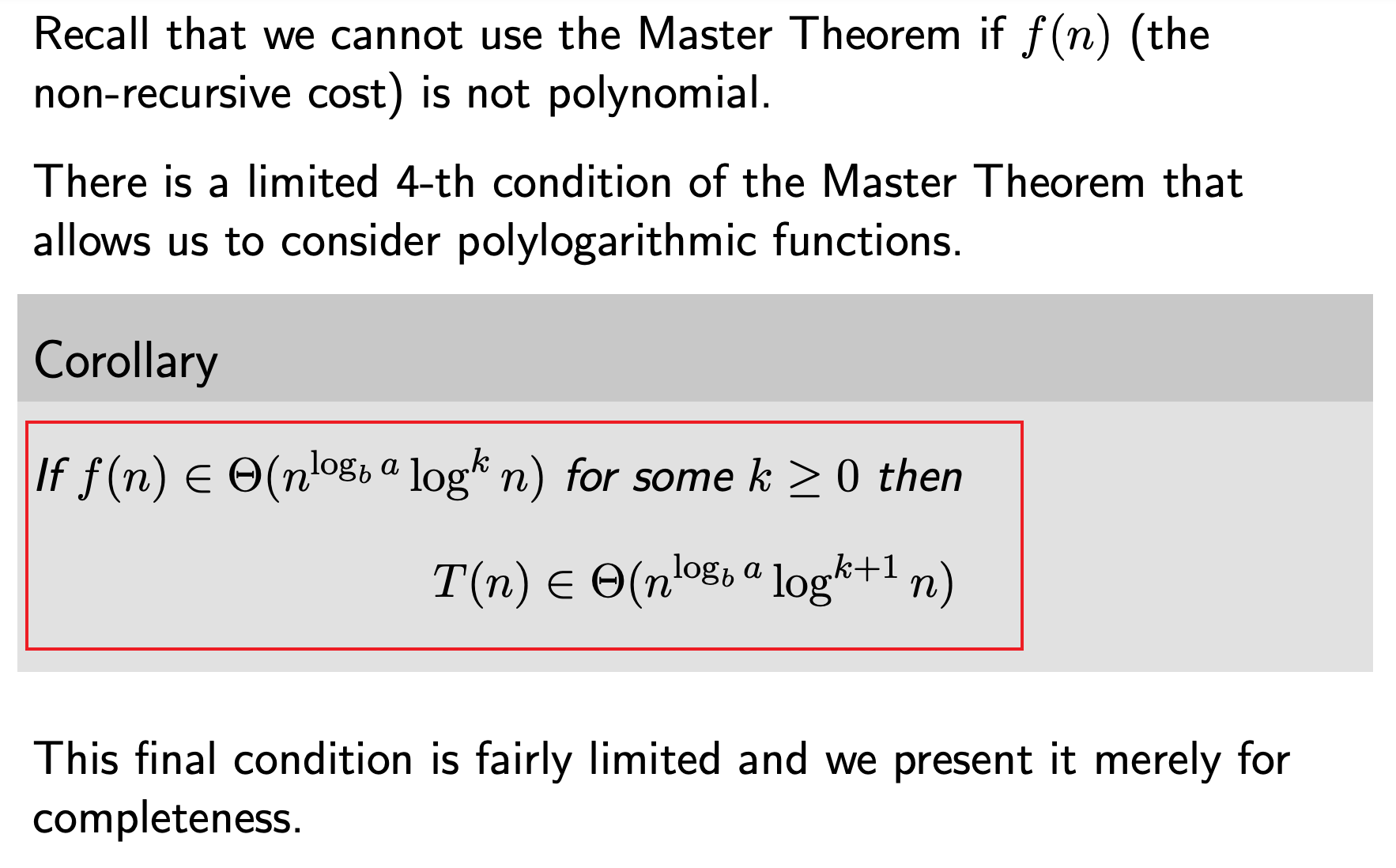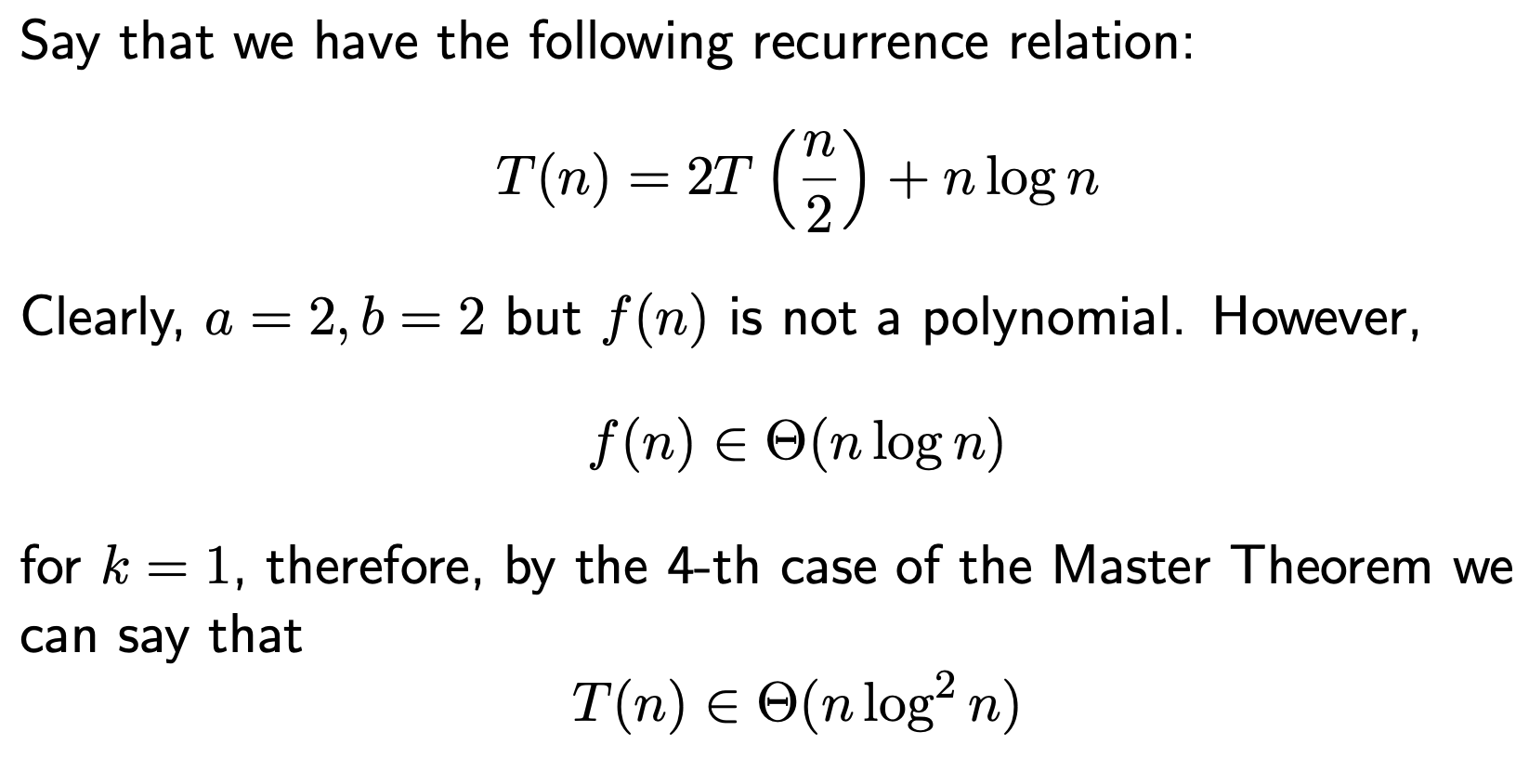# 主定理的内容

《算法导论》中提到了主定理，用来分析分治方法带来的

$$T(n) = aT(n/b) + O(n^d) （a >= 1 且 b > 1）$$

• 如果$a < b^d$，则$T(n) = O(n^d)$
• 如果$a = b^d$，则$T(n) = O(n^dlogn)$
• 如果$a > b^d$，则$T(n) = O(n^{log_b^a})$

# 特殊情况f(n) = logn

$$T(n) = aT(n/b) + f(n)（a >= 1 且 b > 1）$$
Master’s theorem with $f(n)=logn$
MasterTheorem.pdfExample# 具体应用

$T(n) = T(n/2) + O(n^0)$ 假设每个子问题的规模基本一样

$a = 1, b = 2, d = 0,b^d = 1$ ，所以 $T(n) = O(logn)$

$T(n) = 2T(n/2) + O(n^1)$ 假设每个子问题的规模基本一样

$a = 2, b = 2, d = 1,b^d = 2$ ，所以 $T(n) = O(nlogn)$

LeetCode 题解 | 236. 二叉树的最近公共祖先（经典递归 C++）

class Solution {
public:
TreeNode* lowestCommonAncestor(TreeNode* root, TreeNode* p, TreeNode* q) {
if(root == NULL)
return NULL;

// 可认为左右子树已经实现了函数的功能
TreeNode* left =  lowestCommonAncestor(root->left, p, q);
TreeNode* right = lowestCommonAncestor(root->right, p, q);

if(root == p || root == q)
return root;

if(left == NULL)
return right;
if(right == NULL)
return left;
if(left && right) // p和q在两侧
return root;

return NULL; // 必须有返回值
}
};

$T(n) = 2T(n/2) + O(n^0)$ 假设每个子问题的规模基本一样，也就是说我们可以假设左右子树的结点数相同

$a = 2, b = 2, d = 0,b^d = 1$ ，所以 $T(n) = O(n)$

LeetCode 题解 | 105. 从前序与中序遍历序列构造二叉树（递归 C++）# 主定理证明

Author:
Reprint policy: All articles in this blog are used except for special statements CC BY 4.0 reprint polocy. If reproduced, please indicate source SHWEI !

PreviousGitHub中Pull Request的具体过程详解
Pull Request 简单明了的解释“有一个仓库，叫Repo A。你如果要往里贡献代码，首先要Fork这个Repo，于是在你的Github账号下有了一个Repo A2,。然后你在这个A2下工作，Commit，push等。然后你希望原始仓
2020-07-24
Next卡特兰数(Catalan number)定义、证明及例题

2019-10-21
TOC